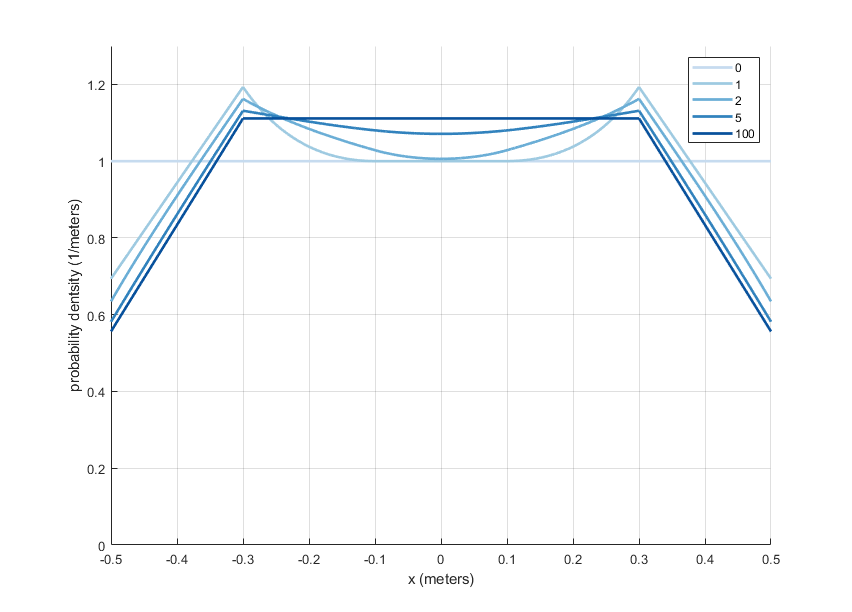# Catch the grasshopperThis week’s Riddler classic is a probability problem about a grasshopper!

You are trying to catch a grasshopper on a balance beam that is 1 meter long. Every time you try to catch it, it jumps to a random point along the interval between 20 centimeters left of its current position and 20 centimeters right of its current position. If the grasshopper is within 20 centimeters of one of the edges, it will not jump off the edge. For example, if it is 10 centimeters from the left edge of the beam, then it will randomly jump to anywhere within 30 centimeters of that edge with equal probability (meaning it will be twice as likely to jump right as it is to jump left). After many, many failed attempts to catch the grasshopper, where is it most likely to be on the beam? Where is it least likely? And what is the ratio between these respective probabilities?

My solution:
[Show Solution]

## One thought on “Catch the grasshopper”

1.MarkS says:

This problem can be solved more quickly by using the principle of detailed balance, which says that the limiting distribution p(y) satisfies Psi(x,y)p(y) = Psi(y,x)p(x).
This implies that Psi(x,y) must take the form F(x,y)/p(y), where F(x,y)=F(y,x). The integration limits in your second integral formula can be rewritten a product of step functions of |x-y| in the integrand, which we then identify as F(x,y), up to overall normalization. This then implies that the denominator in your second integral formula must be p(y), up to overall normalization.